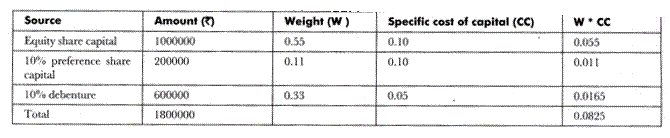# Weighted Average Cost of Capital (WACC)

Weighted average cost of capital is determined by multiplying the cost of each source of capital with its respective proportion in the total capital. Let us understand the concept of weighted average cost of capital with the help of an example. Suppose an organization raises capital by issuing debentures and equity shares.

It pays interest on debt capital and dividend on equity capital. When the organization adds the total interest paid on debt capital to the total dividend paid on equity capital, it obtains weighted average cost of capital. An organization requires generating the profit on its various investments equal to the weighted average cost of capital.

Weighted average cost of capital can be calculated mathematically by using the following formula

Weighted Average Cost of Capital = (KE * E) + (KP * P) + (KD * D) + (KR* R)

Where,

E = Proportion of equity capital in capital structure

P = Proportion of preference capital in capital structure

D = Proportion of debt capital in capital structure

KR = Cost of proportion of retained earnings in capital structure

R = Proportion of retained earnings in capital structure

Let us understand the weighted average cost of capital with the help of some examples.

Example-1

ABC Ltd. has the following capital structure on 31st March 2008

Equity shares (10000 shares issued) = Rs. 1000000

10% preference shares = Rs. 200000

10% debentures = Rs. 600000

The shares of the organization are currently sold at Rs. 100. It is expected that the organization would provide dividend of Rs. 10 per share, which would grow at the rate of 10% forever. Assuming 50% corporate tax rate, calculate weighted average cost of capital on existing capital structure.

Solution:-

The solution is given as follows

Cost of debt after tax = [(1 – T) * R] * 100

KD = [(1-0.50)* 10%] * 100

= 5%

Cost of equity capital when growth rate is given = [(D/MP) + G] * 100

KE = [(10/100) + 10%]* 100

= 10%

Calculation of weighted average cost of capital is shown as followsTherefore, weighted average cost of capital = 0.0825

## 2 thoughts on “Weighted Average Cost of Capital (WACC)”

error: Content is protected !!